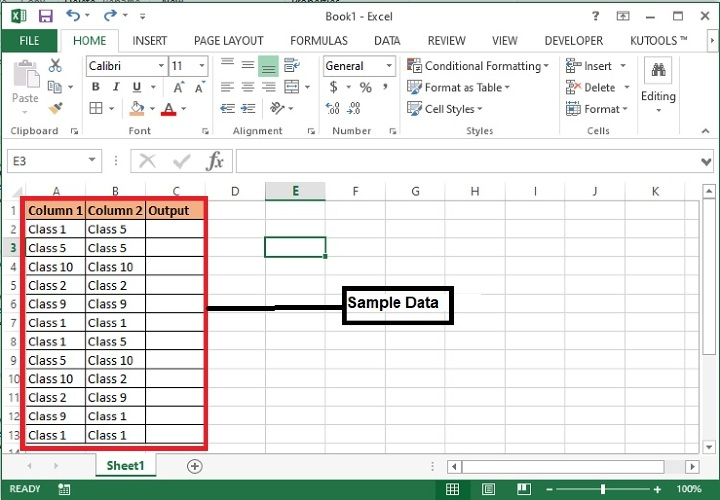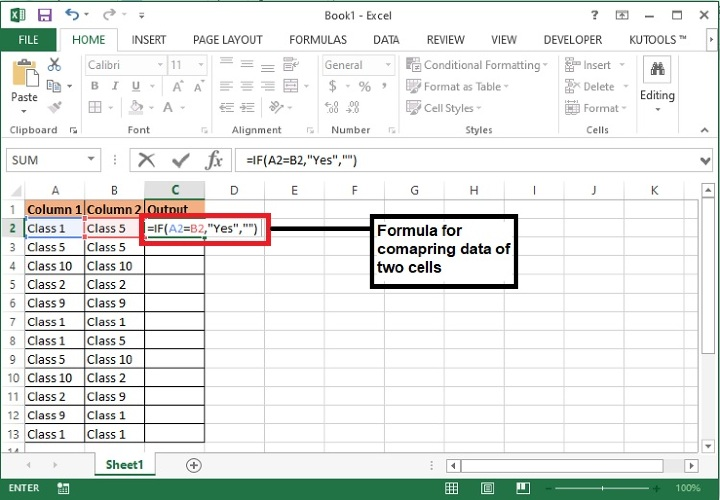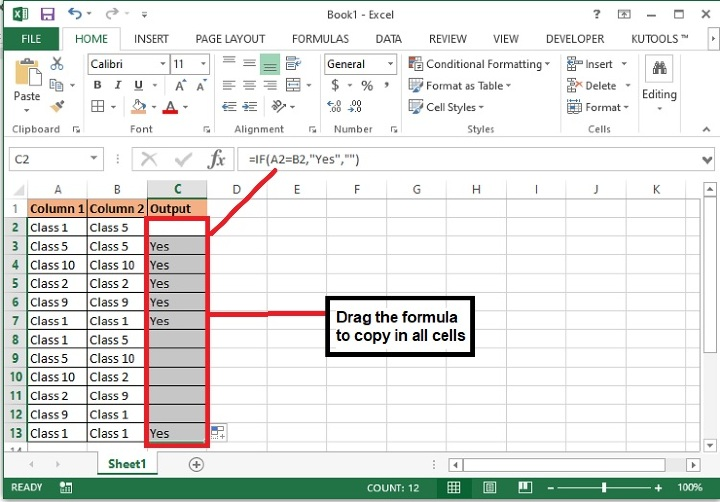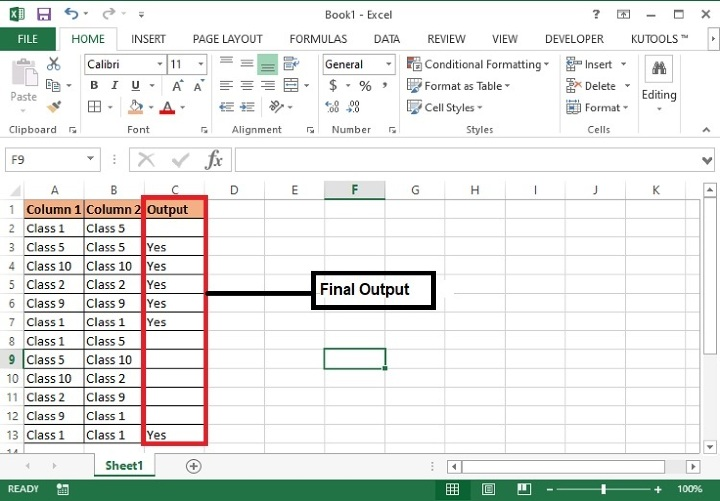# How to Compare Two Cells and Return Yes If They Are Matched In Excel?

When we have a bunch of data and need to compare rows and columns to maintain uniqueness then we might need to apply multiple formulas to the dataset. For instance you have two or more columns and want to compare the values of each row of all columns and return Yes or True of they get matched completely. This can be achieved using the below steps.

## Compare two cells of two columns and return yes if they are perfectly Matched using Formula

Step 1: We have taken the following sample data with some random details. After comparing each row of both the columns system will return yes for the identical match.Step 2: Now, enter the following formula in the cell C2 and drag the same till C13 to copy the formula till the end of the list.

=IF(A2=B2, “Yes”,””)


Here, A2 and B2 is the cell location whose values to be compared with each other. If found true, then system will return Yes otherwise blank. You can add any value in place of Yes and blank as per your choice for example, True, False/Matched, Not matched etc.### Formula Syntax Description

Argument Description
IF(logical_test, {value_if_true},{value_if_false})

Logical_test specifies the condition basis which the data needs to be rendered.

Value_if_true specifies the value that shall be returned if the condition satisfies.

Value_if_false specifies the value that shall be returned if the condition does not satisfy.

Step 3: The system will display “Yes” for the identical values and blank for other values as shown in the screenshot.## Conclusion

Similarly two more rows can be compared using the same formula and adding row location in the formula as =IF(A2=B2=C2=D2, “Yes”,”No”) and so on. This formula can be used to compare any kind of values for example, integers, characters, decimals etc. Keep exploring and keep learning excel.

Updated on: 29-Aug-2023

40 Views